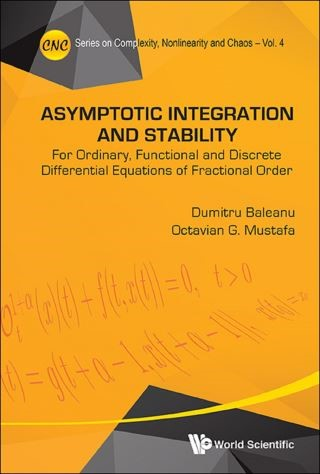## Asymptotic Integration And Stability: For Ordinary, Functional And Discrete Differential Equations Of Fractional Order• Description
• Author
• Info
• Reviews

### Description

This volume presents several important and recent contributions to the emerging field of fractional differential equations in a self-contained manner. It deals with new results on existence, uniqueness and multiplicity, smoothness, asymptotic development, and stability of solutions. The new topics in the field of fractional calculus include also the Mittag-Leffler and Razumikhin stability, stability of a class of discrete fractional non-autonomous systems, asymptotic integration with a priori given coefficients, intervals of disconjugacy (non-oscillation), existence of Lp solutions for various linear, and nonlinear fractional differential equations.

Contents:
• The Differential Operators of Order 1 + α and Their Integral Counterparts
• Existence and Uniqueness of Solution for the Differential Equations of Order α
• Position of the Zeros, the Bihari Inequality, and the Asymptotic Behavior of Solutions for the Differential Equations of Order α
• Asymptotic Integration for the Differential Equations of Order 1 + α
• Existence and Uniqueness of Solution for Some Delay Differential Equations with Caputo Derivatives
• Existence of Positive Solutions for Some Delay Fractional Differential Equations with a Generalized N-Term
• Stability of a Class of Discrete Fractional Nonautonomous Systems
• Mittag-Leffler Stability for Fractional Nonlinear Systems with Delay
• Razumikhin Stability for Fractional Systems in the Presence of Delay
• Controllability of Some Fractional Evolution Nonlocal Impulsive Quasilinear Delay Integro-Differential Systems
• Approximate Controllability of Sobolev Type Nonlocal Fractional Stochastic Dynamic Systems in Hilbert Spaces

Readership: Researchers and PhD students in mathematics, physics and applied sciences whose interests involve differential equations, fractional calculus, special functions, variational methods and their applications in physics, mechanics, engineering, economics, life sciences and social sciences.
Key Features:
• An introductory part saves the readers from having to delve into the more specialized literature on fractional calculus
• Self-contained with fully detailed proofs of new technical results
• Includes various open problems along with ways of approaching their solutions
• The authors are known experts in the topics of fractional calculus as well as their applications
• Contains materials which complement existing books on fractional calculus